﻿ DefaultParticleSystemMagnet.DistanceFunctions Enumeration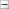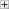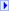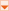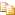Collapse AllExpand AllCode: All Code: Multiple Code: C# Code: Visual Basic Code: Visual C++

[TCheckBoxFormControlObj]C#

[TCheckBoxFormControlObj]Visual Basic

[TCheckBoxFormControlObj]
Visual C++
 DPSF API Documentation DefaultParticleSystemMagnet . . :: ..DistanceFunctions EnumerationFunction used to determine how much the Magnet should affect a Particle, based on how far away the Particle is from the Magnet.

Namespace:

Assembly: DPSF (in DPSF.dll) Version: 2.5.0.0Syntax

 C# [SerializableAttribute] public enum DistanceFunctions
 Visual Basic Public Enumeration DistanceFunctions
 Visual C++ [SerializableAttribute] public enum class DistanceFunctionsMembers

 Member name Value Description Constant 0 As long as the Particle's distance from the Magnet is between the Min and Max Distance of the Magnet, the Max Force will be applied to the Particle. Function Logic: y = 1, where y is the normalized Force applied and 1 is the Particle's normalized distance between the Magnet's Min and Max Distances. Linear 1 The Force applied to the Particle will be Linearly interpolated from zero to Max Force based on the Particle's distance from the Magnet between the Magnet's Min and Max Distances. So when the Particle is at the Min Distance from the Magnet no force will be applied, when the Particle is at half of the Max Distance from the Magnet 1/2 of the Max Force will be applied, and when the Particle is at the Max Distance from the Magnet the Max Force will be applied to the Particle. Function Logic: y = x, where y is the normalized Force applied and x is the Particle's normalized distance between the Magnet's Min and Max Distances. Squared 2 The Force applied to the Particle will be Squared interpolated from zero to Max Force based on the Particle's distance from the Magnet between the Magnet's Min and Max Distances. So when the Particle is at the Min Distance from the Magnet no force will be applied, when the Particle is at half of the Max Distance from the Magnet 1/4 of the Max Force will be applied, and when the Particle is at the Max Distance from the Magnet the Max Force will be applied to the Particle. Function Logic: y = x * x, where y is the normalized Force applied and x is the Particle's normalized distance between the Magnet's Min and Max Distances. Cubed 3 The Force applied to the Particle will be Cubed interpolated from zero to Max Force based on the Particle's distance from the Magnet between the Magnet's Min and Max Distances. So when the Particle is at the Min Distance from the Magnet no force will be applied, when the Particle is at half of the Max Distance from the Magnet 1/8 of the Max Force will be applied, and when the Particle is at the Max Distance from the Magnet the Max Force will be applied to the Particle. Function Logic: y = x * x * x, where y is the normalized Force applied and x is the Particle's normalized distance between the Magnet's Min and Max Distances. LinearInverse 4 The Inverse of the Linear function. That is, when the Particle is at the Min Distance from the Magnet the Max Force will be applied, when the Particle is at half of the Max Distance from the Magnet 1/2 of the Max Force will be applied, and when the Particle is at the Max Distance from the Magnet no force will be applied to the Particle. SquaredInverse 5 The Inverse of the Squared function. That is, when the Particle is at the Min Distance from the Magnet the Max Force will be applied, when the Particle is at half of the Max Distance from the Magnet 1/4 of the Max Force will be applied, and when the Particle is at the Max Distance from the Magnet no force will be applied to the Particle. CubedInverse 6 The Inverse of the Cubed function. That is, when the Particle is at the Min Distance from the Magnet the Max Force will be applied, when the Particle is at half of the Max Distance from the Magnet 1/8 of the Max Force will be applied, and when the Particle is at the Max Distance from the Magnet no force will be applied to the Particle.See Also

DPSF NamespaceSend comments on this topic to